# Creating Visualizations

Typically, a function that produces a plot in R performs the data crunching and the graphical rendering. For example, `geom_histogram()` calculates the bin sizes and the count per bin, and then it renders the plot. Plotting functions usually require that 100% of the data be passed to them. This is a problem when working with a database. The best approach is to move the data transformation to the database, and then use a graphing function to render the results.

• Demonstrate a practical implementation of the “Transform in database, plot in R” concept by showing how to visualize a categorical variable using a Bar plot, a single continuous variable using a Histogram, and two continuous variables using a Raster plot, all using data in a database

• Introduce a technique that simplifies the use of complex formulas that are required to move the calculations of the plot to the database

An alternative, is to use a helper R package that already implements the principles shared in this article.

## Bar plot

A Bar plot is intended to measure and compare categorical data. Passing the category to `geom_bar()` as `x` will automatically calculate the height of the bars based on the row count per category. Here is the code of a typical bar plot using `ggplot2`:

``````ggplot(data = flights) +
geom_bar(aes(x = origin), stat = "count")``````

### Data transformation

Because `dplyr` is being used to compute the count per category inside the database, the discrete values are separated using `group_by()`, followed by `tally()` to obtain the row count per category. Lastly, `collect()` downloads the results into R:

``````
df <- tbl(con, "flights") %>%
group_by(origin) %>%
tally() %>%
collect()

df``````
``````## # A tibble: 3 x 2
##   origin      n
##   <chr>   <int>
## 1 LGA    104662
## 2 EWR    120835
## 3 JFK    111279``````

### Plotting results in R

The results of the Data Transformation step can now be used in `ggplot2` to render the plot. This time, `geom_col()` is used instead of `geom_bar()` because the height of the bars have been pre-calculated by `dplyr`:

``````ggplot(data = df) +
geom_col(aes(x = origin, y = n)) ``````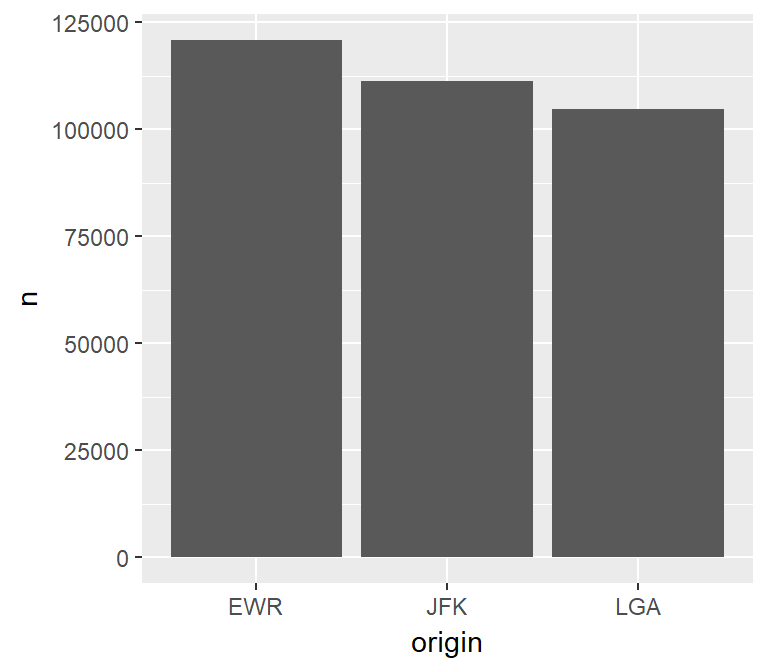### Transform and plot

The plot can be created using a single piped line of code. This is particularly useful when performing exploratory data analysis because it is easy to add or remove filters, or to change the variable that is being analyzed.

``````tbl(con, "flights") %>%
group_by(origin) %>%
tally() %>%
collect() %>%
ggplot() +
geom_col(aes(x = origin, y = n)) ``````## Histogram

The histogram is intended to visualize the distribution of the values of a continuous variable. It does this by grouping the values into bins with the same range of values. In essence, a histogram converts a continuous variable to a discrete variable by splitting and placing the variable’s values into multiple bins.

### Calculations

The following breakdown of the calculation needed to create a histogram is intended to highlight the complexity of moving its processing to the database.

For example, if a histogram with 20 bins is needed, and the variable has a minimum value of 1 and a maximum value of 101, then each bin needs to be 5.

• 101 (Max value) - 1 (Min value) = 100
• 100 / 20 (Number of bins) = 5

The first bin will have a range of 1 to 6, the second 7 to 12, etc.

After that, the count of values that are inside each range needs to be determined. In this example, there may be two rows that have a value between 1 and 6 and five rows with values between 7 and 12.

Any formula used to create a Histogram will need to calculate the bins, place the values inside the bins, and only call math functions supported by the database in use.

### Using a helper function

An advantage of using `dplyr` to convert the continuous variable into a discrete variable is that one solution can be applied to multiple database types. This is possible if the resulting formula is made of basic functions that most SQL databases support and is expressed in R, so that `dplyr` can translate it into the proper SQL syntax.

Unfortunately, the formula is rather long and mistakes can be made if used in multiple locations, because any corrections to the formula may not be propagated to all of the instances. To solve this, a helper function can be used.

In the following helper function, the `var` input is used to build the formula in an unevaluated R code format. When used inside `dplyr`, it will return the assembled formula which will then be evaluated as inside the verb command. Feel free to copy this function into your script or R Notebook.

The function has two other arguments:

• `bins` - this allows the number of bins to be customized. It defaults to 30
• `binwidth` - this is used to specify the size of the bin. It overrides any value passed to the `bins` argument.
``````library(rlang)
db_bin <- function(var, bins = 30, binwidth = NULL) {
var <- enexpr(var)

range <- expr((max(!! var, na.rm = TRUE) - min(!! var, na.rm = TRUE)))

if (is.null(binwidth)) {
binwidth <- expr((!! range / !! bins))
} else {
bins <- expr(as.integer(!! range / !! binwidth))
}

# Made more sense to use floor() to determine the bin value than
# using the bin number or the max or mean, feel free to customize
bin_number <- expr(as.integer(floor((!! var - min(!! var, na.rm = TRUE)) / !! binwidth)))

# Value(s) that match max(x) will be rebased to bin -1, giving us the exact number of bins requested
expr(((!! binwidth) *
ifelse(!! bin_number == !! bins, !! bin_number - 1, !! bin_number)) + min(!! var, na.rm = TRUE))
}``````

Notice that the function returns a quosure containing the unevaluated R code that calculates the bins. To read more about how this kind of approach works, please refer to this article: Programming with dplyr.

It is important to note that the database in use needs to support the functions called in the formula, such as `min()` and `max()`.

Here is an example of the function’s output. Notice that a fictitious field called `any_field` is used, and no “missing field” error is generated. That is because the formula has not yet been evaluated.

``db_bin(any_field)``
``````## (((max(any_field, na.rm = TRUE) - min(any_field, na.rm = TRUE))/30) *
##     ifelse(as.integer(floor((any_field - min(any_field, na.rm = TRUE))/((max(any_field,
##         na.rm = TRUE) - min(any_field, na.rm = TRUE))/30))) ==
##         30, as.integer(floor((any_field - min(any_field, na.rm = TRUE))/((max(any_field,
##         na.rm = TRUE) - min(any_field, na.rm = TRUE))/30))) -
##         1, as.integer(floor((any_field - min(any_field, na.rm = TRUE))/((max(any_field,
##         na.rm = TRUE) - min(any_field, na.rm = TRUE))/30))))) +
##     min(any_field, na.rm = TRUE)``````

This is an example of the function using `binwidth`. The resulting formula is a little different.

``db_bin(any_field, binwidth = 300)``
``````## (300 * ifelse(as.integer(floor((any_field - min(any_field, na.rm = TRUE))/300)) ==
##     as.integer((max(any_field, na.rm = TRUE) - min(any_field,
##         na.rm = TRUE))/300), as.integer(floor((any_field - min(any_field,
##     na.rm = TRUE))/300)) - 1, as.integer(floor((any_field - min(any_field,
##     na.rm = TRUE))/300)))) + min(any_field, na.rm = TRUE)``````

### Data transformation

The data processing is very simple when using the helper function. The `db_bin` function is used inside `group_by()`. There are a couple of must-do’s to keep in mind:

• Specify the name of the field that uses the `db_bin()` function - If a name is not specified, `dplyr` will use the long formula text as the default name of the field, which in most cases breaks the database’s field name length rules.

• Prefix `!!` to the `db_bin()` function - This triggers the processing, or evaluation, of the function, which returns the complex formula.

``````df <- tbl(con, "flights") %>%
group_by(x = !! db_bin(sched_dep_time, bins = 10)) %>%
tally() %>%
collect()

``````## # A tibble: 6 x 2
##       x     n
##   <dbl> <int>
## 1  782. 51999
## 2  557. 48864
## 3 1007. 38889
## 4  106.     1
## 5  331.  1861
## 6 1908. 39942``````

### Plotting results in R

Because the bins have been pre-processed on and collected from the database, the results are easily plotted using `geom_col()`. The resulting bin values are `x` and the count per bin is `y`:

``````ggplot(data = df) +
geom_col(aes(x = x, y = n))``````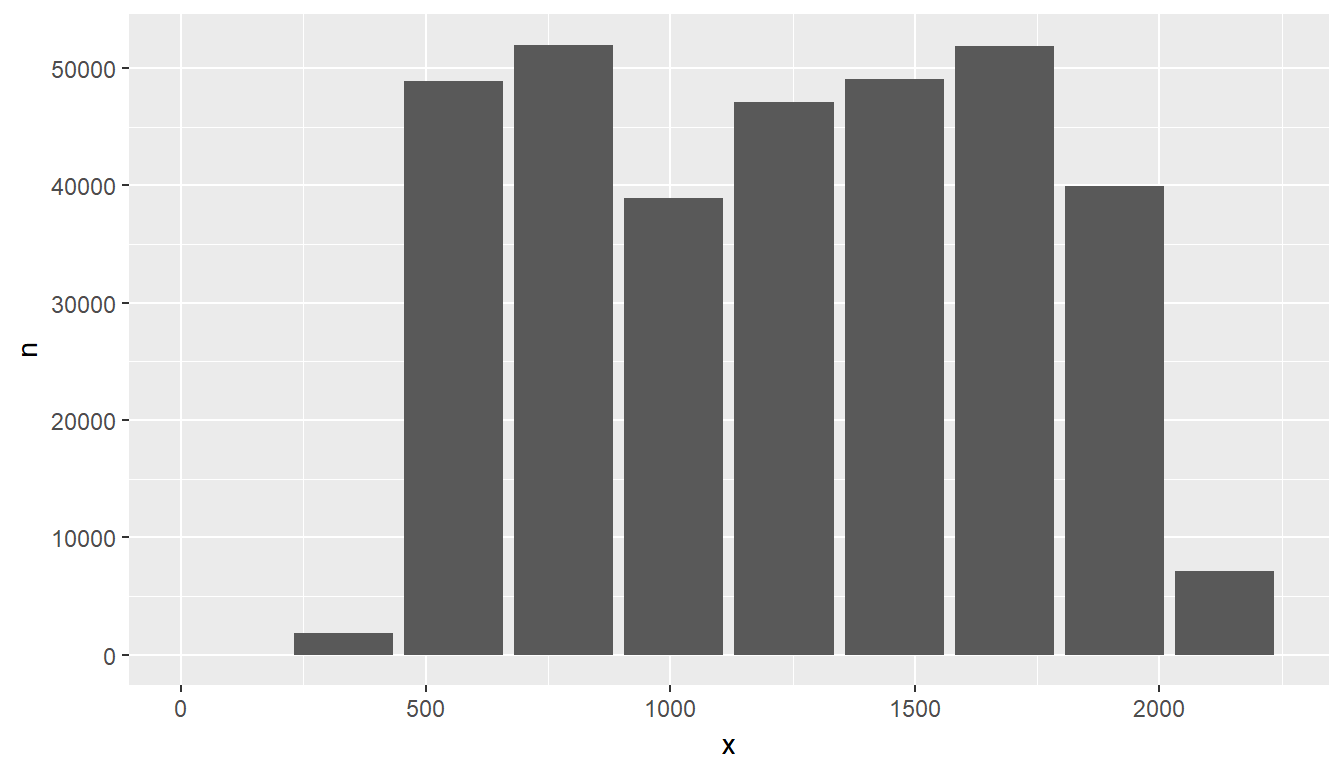### Transform and plot

Just like with the Bar plot, the entire process can be piped. Here is an example of using the `binwidth` argument instead of `bins`; additionally, the bin size is widened to 300-minute intervals:

``````tbl(con, "flights") %>%
group_by(x = !! db_bin(sched_dep_time, binwidth = 300)) %>%
tally() %>%
collect() %>%
ggplot() +
geom_col(aes(x = x, y = n))
``````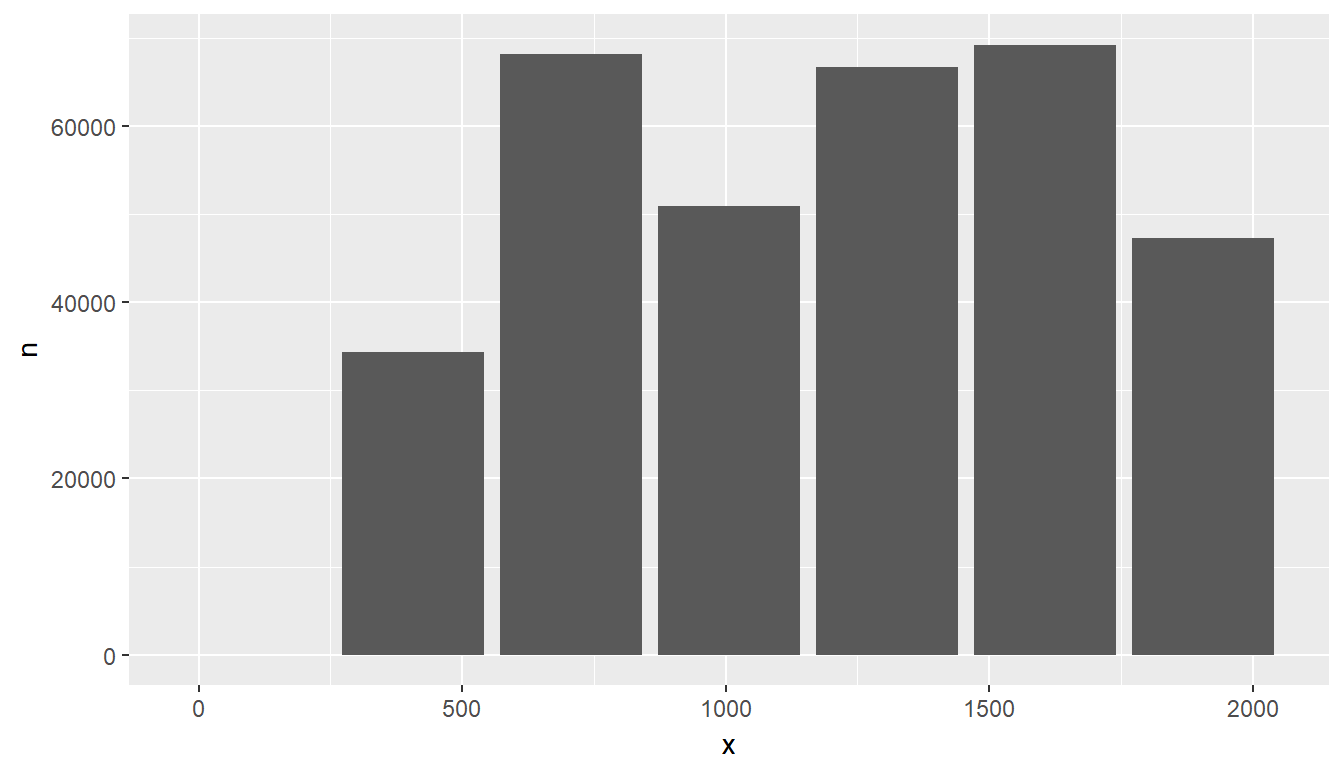## Raster Plot

To visualize two continuous variables, we typically resort to a Scatter plot. However, this may not be practical when visualizing millions or billions of dots representing the intersections of the two variables. A Raster plot may be a better option, because it concentrates the intersections into squares that are easier to parse visually.

A Raster plot basically does the same as a Histogram. It takes two continuous variables and creates discrete 2-dimensional bins represented as squares in the plot. It then determines either the number of rows inside each square or processes some aggregation, like an average.

### Data transformation

The same helper function used to create the Histogram can be used to create the squares. The `db_bin()` function is used for each continuous variable inside `group_by()`, but in this case the number if `bins` is increased to 50:

``````df <- tbl(con, "flights") %>%
group_by(
sc_dep_time = !! db_bin(sched_dep_time, bins = 50),
sc_arr_time = !! db_bin(sched_arr_time, bins = 50)
) %>%
summarise(avg_distance = mean(distance)) %>%
collect()

``````## # A tibble: 6 x 3
## # Groups:   sc_dep_time 
##   sc_dep_time sc_arr_time avg_distance
##         <dbl>       <dbl>        <dbl>
## 1       1953.       2170.         596.
## 2       2044.       2123.         201.
## 3       1728.       1887.         496.
## 4       1863.       2076.         687.
## 5       1638.       1793.         422.
## 6        737.        944.         593.``````

### Plotting results in R

The plot can now be built using `geom_raster()`. Assigning `x` and `y` to each of the continuous variables will depend on what makes more sense for a given visualization. The result of each intersection is passed as the color of the square using `fill`.

``````ggplot(data = df) +
geom_raster(aes(x = sc_dep_time, y = sc_arr_time, fill = avg_distance))``````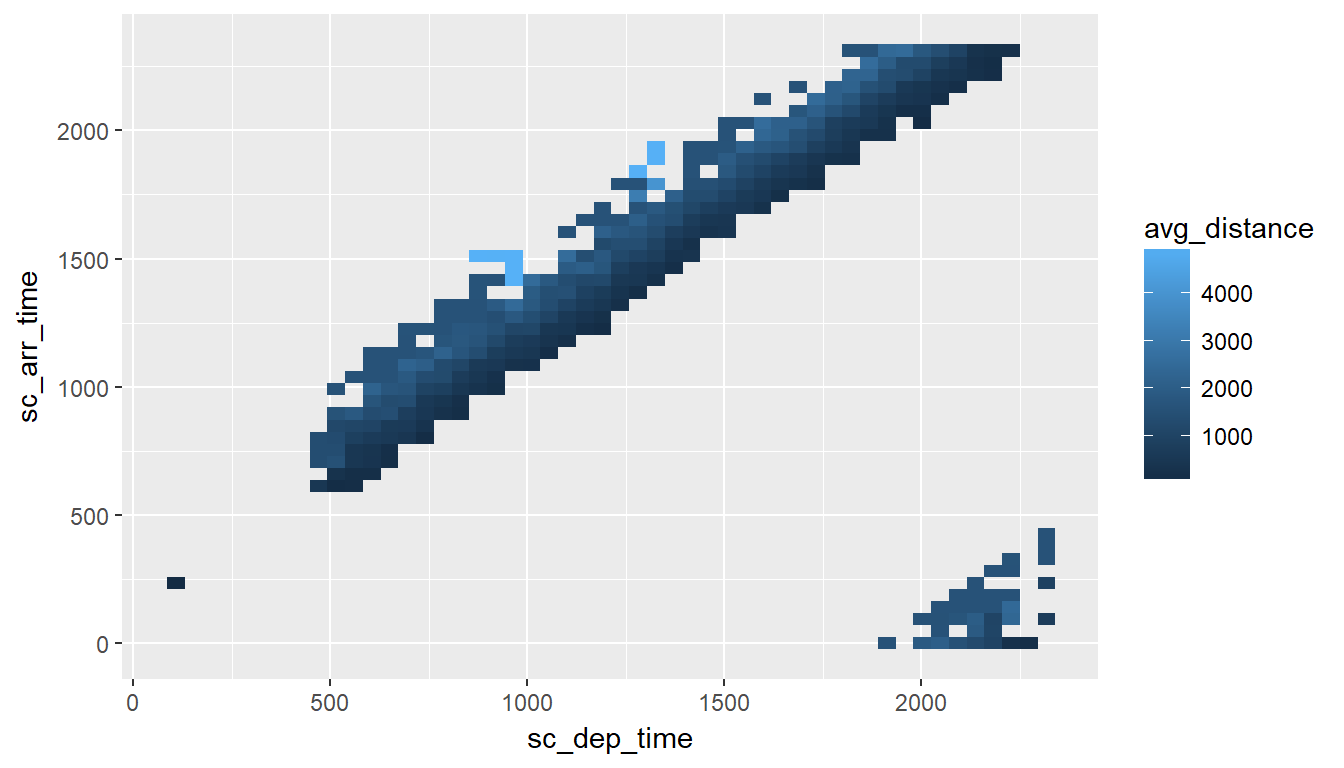### Considerations

There are two considerations when using a Raster plot with a database. Both considerations are related to the size of the results downloaded from the database:

• The number of `bins` requested: The higher the `bins` value is, the more data is downloaded from the database.

• How concentrated the data is: This refers to how many intersections return a value. The more intersections without a value, the less data is downloaded from the database.

In the previous example, there is a maximum of 2,500 rows (50 x 50). Because the data is highly concentrated, only 353 records are returned. This means that the data will be transmitted over the network quickly, but the trade-off is that the picture definition may not be ideal to gain insights about the data.

In the following example, the “definition” is set at 100 x 100. This improves the resolution but it quadruples the number of records that could potentially be downloaded.

``````tbl(con, "flights") %>%
group_by(
sc_dep_time = !! db_bin(sched_dep_time, bins = 100),
sc_arr_time = !! db_bin(sched_arr_time, bins = 100)
) %>%
summarise(avg_distance = mean(distance)) %>%
collect() %>%
ggplot() +
geom_raster(aes(x = sc_dep_time, y = sc_arr_time, fill = avg_distance))``````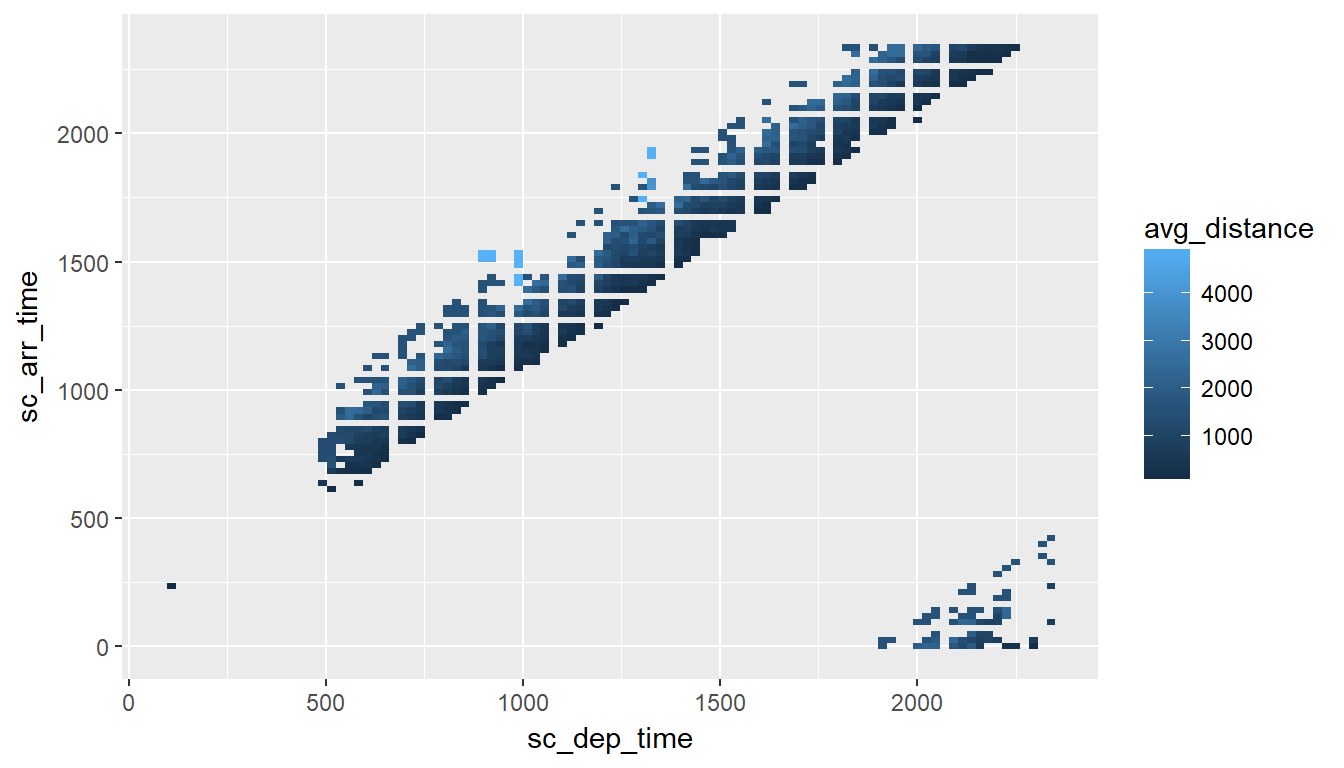## Use an R package

The dbplot package provides helper functions that automate the aggregation and plotting steps. For more info, visit the dbplot site.